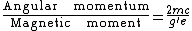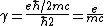# Gyromagnetic Ratio

Also found in: Dictionary, Medical, Wikipedia.

## Gyromagnetic ratio

The ratio of angular momentum to magnetic moment for atomic systems. This ratio is usually expressed in terms of the magnetomechanical factor g, as in Eq. (1).

(1)The ratio is written here in electromagnetic units; thus, e/c and m are the charge and mass of the electron. The factor g is sometimes loosely called the gyromagnetic ratio.

The magnetomechanical ratio is the inverse of the gyromagnetic ratio. It is usually denoted by γ and is equal to ge/2mc. The magnetomechanical ratio of a substance identifies the origin of the magnetic moment. For example, for electron spin the angular momentum is ½&planck;, where &planck; is Planck's constant divided by 2π. The magnetic moment is the Bohr magneton e&planck;/2mc. Thus, the magnetomechanical ratio

(2)is given by Eq. (2). Since γ = ge/2mc, for electron spin g = 2. For orbital angular momentum, γ = e/mc and g = 1. The experimental values of g for most ferromagnetic materials are in the neighborhood of 2, showing that the major contribution to the magnetization comes from the electron spin. In superconductors, on the other hand, the fact that g = 1 shows that the diamagnetic currents which cause the Meissner effect are caused by electrons. See Meissner effect, Superconductivity

## Gyromagnetic Ratio

the ratio of the magnetic moment of elementary particles and systems of elementary particles, such as atoms, molecules, and atomic nuclei, to their angular momentum (mechanical moment). The gyromagnetic ratio has a definite value for each elementary particle with nonzero mechanical momentum, or spin. The values may be determined for various states of an atomic system from the equation γ = gγo, where γo is the unit of the gyromagnetic ratio and is the Lande factor. In this case, the unit of the gyromagnetic ratio is its value for the orbital motion of the electron in an atom, —e/2meC, where e is the magnitude of the elementary electric charge, me is the mass of an electron, and c is the speed of light. The gyromagnetic ratio for nuclei is the analogous quantity for the proton in a nucleus: e/mpC, where mp is the mass of a proton.

The value of the gyromagnetic ratio determines the effect of magnetic fields on a system that has a magnetic moment. According to classical theory, the magnetic moment in an external magnetic field of intensity H undergoes precession, which is uniform rotation about the direction of H, with the preservation of the angle of inclination, at an angular velocity ω = — γH. In a special case, in which the magnetic moment is due to the orbital motion of the electrons, Larmor precession results. The characteristic value of magnetic splitting of the energy levels in a magnetic field is determined by the gyromagnetic ratio according to quantum theory. In this case the value is γhH = ohH, where h is Planck’s constant.

M. A. EL’IASHEVICH

## gyromagnetic ratio

[¦jī·rō·mag′ned·ik ′rā·shō]
(physics)
The ratio of the magnetic dipole moment to the angular momentum for a classical, atomic, or nuclear system.
Occasionally, the reciprocal of the quantity in the first definition.
References in periodicals archive ?
Nonlinear electrodynamics coupled to gravity and satisfying the weak energy condition admits the class of regular solutions describing spinning charged soliton asymptotically Kerr-Newman for a distant observer with a gyromagnetic ratio g = 2.
Here [Gamma] is the gyromagnetic ratio, g is the gradient amplitude, and [Delta] is the gradient duration.
(ii) the efficiency of the gradient which, in practice, is lowered by a factor of 4 (with respect to proton measurements), because it acts through the expression [Gamma]g, where g is the gradient amplitude (independent of the observed nuclei in the case of static field gradients) and [Gamma] the gyromagnetic ratio.
The permeability tensor is dependent upon the material's saturation magnetization and its gyromagnetic ratio, which are derived by considering the origin of the ferrite's magnetic moments.
A measurement of the gyromagnetic ratio of the proton by the strong field method, Atomic Masses and Fundamental Constants, J.
Ed Williams has worked on various projects at NIST, including determining the low field gyromagnetic ratio of the proton.
The Electricity and Optics Division began preparations for measuring the fundamental constant [[gamma].sub.p], the gyromagnetic ratio of the proton, which would relate the measurement of magnetic field strength to the nuclear magnetic resonance (NMR) frequency of the proton.
Olsen, New measurement of the proton gyromagnetic ratio and a derived value of the fine-structure constant, Phys.
[Mathematical Expression Omitted] where [Delta][Eta] = the ferromagnetic resonance linewidth [GAMMA] = the gyromagnetic ratio = 2.8 MHz/Oe Linewidths ([GAMMA][ETA]) as small as 0.3 Oe are achievable in epitaxial YIG films, making feasible the fabrication of narrowband filters with very low loss.
In this equation, [gamma] is the gyromagnetic ratio defined as e/m = 2.8 MHz/Oe, where e and m are the magnitude of the charge and the mass of an electron.

Site: Follow: Share:
Open / Close